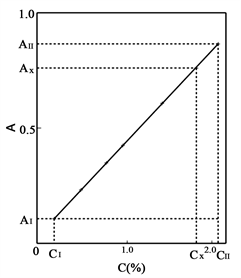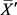﻿ 对光度法的几点新认识

# 对光度法的几点新认识Some New Understandings on Spectrophotometry

Abstract: Taking the spectrophotometric determination of manganese in carbon steel, high manganese steel and ferromanganese as an example, seven new understandings of photometry are introduced under the condition of strictly following the “precision rule”, i.e. “to maintain infection consistency of each factor for each sample (including standard sample) in the same measurement series”: 1) The “calibration curve” interval of the method is actually the domain of the incident light. In this interval, the difference ΔA of absorbance is proportional to the difference ΔC of the matter that absorbs light: ΔA = KΔC , not A = KC; 2) The reason for the discrepancy between the photometric method and Lambert-Beer’s law is not the “non-monochromaticity” of incident light. Photometer should not pursue the monochromaticity of incident light, which should adopt the “non-monochromatic” incident light to improve its sensitivity. 3) The relative error of spectrophotometric results is independent of the transmittance, and the relative error of the optimum measurement range will not be 1%. 4) Within the “calibration curve” of the method, all the sample content and its absorbance coordinate points (C, A) will inevitably fall on the same line of ΔA = KΔC. Therefore, the connection between the upper and lower coordinate points of the standard sample is the “determination line” of the sample content, without the need to draw “calibration curve” with multi-standard samples. 5) The “absorption band” is the reflect of quantized absorption of different quantum levels in the ground state of chemicals, so its different wavelengths can be used to determine the content of related matter, not just its peak value. 6) The content range given by each method is actually the absorbance range of the incident light with the corresponding content. Samples with different contents can be determined by the original method as long as they are made into samples within the range of incident light absorbance. 7) The uncertainty of photometric determination results:U95=0.67R/√n、kp=2，U99=R/√n、kp=3(R is sample range, n is sample capacity), which are simple, clear and realistic. The new understanding simplifies the operation and uncertainty evaluation of spectrophotometry, expands the determination content of spectrophotometry to any content, and provides a new idea for the development of photometer.

1. 引言

2. 实验部分

2.1. 主要仪器与试剂

2.2. 实验条件

2.2.1. 人的因素控制

2.2.2. 机械因素的控制

2.2.3. 物料因素的控制

2.2.4. 环境因素的控制

2.3. 实验方法

2.3.1. 碳钢中锰的测定

2.3.2. 高锰钢中锰的测定

2.3.3. 锰铁中锰的测定

3. 结果与讨论

3.1. “化学物质吸光规律”Table 1. Absorption of manganese standard sample at 520 nmFigure 1. Calibration curve of Mn

$\left({A}_{x}-{A}_{Ⅰ}\right)/\left({A}_{Ⅱ}-{A}_{Ⅰ}\right)=\left({C}_{x}-{C}_{Ⅰ}\right)/\left({C}_{Ⅱ}-{C}_{Ⅰ}\right)$ (1)

${A}_{Ⅱ}-{A}_{Ⅰ}=k\left({C}_{Ⅱ}-{C}_{Ⅰ}\right)$ (2)

$\Delta A=k\Delta C$ (3)

${C}_{x}=\left[\left({A}_{x}-{A}_{Ⅰ}\right)\left({C}_{Ⅱ}-{C}_{Ⅰ}\right)/\left({A}_{Ⅱ}-{A}_{Ⅰ}\right)\right]+{C}_{Ⅰ}$ (4)

${C}_{x}=\left(1.182/0.746\right)+0.19=1.774\left(%\right)$

3.2. 吸收谱带内的光具有加合性Table 2. Absorption of the same series of high manganese steel at 530 nm of different photometers

3.3. 光度法测定结果的相对误差Table 3. RSD of determination results of samples with different transmittance

3.4. 光度法的测定含量上、下限Table 4. Determination results of high manganese steel and ferromanganese

3.5. 测定结果的不确定度评定

$u\left({\stackrel{¯}{X}}^{\prime }\right)=S/\sqrt{n}$ (5)

${U}_{95}=2S/\sqrt{n}$${k}_{p}=\text{2}$ (6)

${U}_{99}=3S/\sqrt{n}$${k}_{p}=\text{3}$ (7)

S是“小样本”各测得值的实验标准偏差。“小样本”近似正态分布，所以S可按照极差法 $S=R/C$ 评估。根据“规范”要求，“小样本”容量应取10，则 $C\approx \text{3}$$S=R/3$  。将 $S=R/3$ 代入式(2)、式(3)，即可得到量值μ“小样本”均值的扩展不确定度：

${U}_{95}=0.67R/\sqrt{n}$${k}_{p}=\text{2}$ (8)

${U}_{99}=R/\sqrt{n}$${k}_{p}=\text{3}$ (9)

4. 结语

 尚庆坤, 崔运成, 赫春香, 等. 分析化学[M]. 北京: 科学出版社, 2014: 201-208.

 王玉枝, 张正奇, 宦双燕, 等. 分析化学(第三版)[M]. 北京: 科学出版社, 2016: 186-190.

 王文渊, 曲中堂. 分析化学[M]. 北京: 化学工业出版社, 2013: 110-114.

 彭晓文, 程玉红. 分析化学[M]. 北京: 中国铁道出版社, 2014: 126-130.

 王彤, 段春生. 分析化学(第二版)[M]. 北京: 高等教育出版社, 2013: 237-244.

 钟国秀, 黄清华. 光度法快速测定铜合金中微量铝[J]. 化学分析计量, 2011, 20(5): 60-62.

 王冀艳, 刘勉, 赵晓亮, 等. 酸溶–苯基荧光酮光度法测定矿样中钽[J]. 冶金分析, 2017, 37(4): 48-51.

 王璇, 金涛, 王浩伟, 等. 紫外分光光度法测定聚硫密封胶中二氧化钛[J]. 化学分析计量, 2017, 26(4): 24-27.

 高立红, 周凯红, 王燕霞, 等. 硅钼蓝分光光度法测定氟化稀土中二氧化硅[J]. 冶金分析, 2017, 37(4): 57-61.

 邓军华, 王一凌, 亢德华, 等. 二氨替比林甲烷光度法测定含钛物料中二氧化钛[J]. 冶金分析, 2015, 35(10): 30-35.

 袁秉鉴. 高锰酸分光光度法的研究[J]. 化学分析计量, 2006, 15(2): 13-17.

 杨振明. 概率论(第二版)[M]. 北京: 科学出版社, 2008: 74.

 余君武, 肖艳清. 概率论与数理统计[M]. 北京: 理工大学出版社, 2009: 95.

 郑德如. 统计学[M]. 上海: 立信会计出版社, 1994: 174-177.

 林景星, 陈丹英. 计量基础知识(第二版)[M]. 北京: 中国计量出版社, 2008: 152-155.

 JJF1059.1-2012 测量不确定度评定与表示[S].

 袁秉鉴. 光度法不确定度的数学模型[J]. 计量技术, 2010(1): 67-70.

 袁秉鉴. 重复测量结果的不确定度评定[J]. 中国计量, 2015(1): 89-90.

Top